# Stress and Strain

#### description

• Stress
1. Tensile stress or compressive stress
2. Volume stress or hydraulic stress
3.  Shearing stress
• Strain
1. Tensile strain
2. Volume strain
3. Shearing strain

#### notes

Stress & Strain:

stress:-

The internal restoring force setup per unit area of cross-section of the deformed body is called stress.

If a body gets deformed under the action of an external force, then at each section of the body an internal force of the reaction is set up which tends to restore the body into its original state.

"Stress" = "Applied Force"/"Area"

unit: "N"//m^2 or pascal.

Its dimensional formula is ["ML"^-1"T"^-2]

Types of stress:

There are different types of stress

1. Longitudinal stress: if deforming force is applied normal to the area of cross-section, then the stress is called longitudinal stress. It is further categorized into two types.

(a) Tensile stress: if there is an increase in the length of the object under the effect of applied force, then stress is called tensile stress.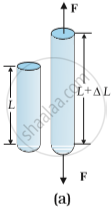(b) Compressional stress: If there is a decrease in the length of the object under the effect of applied force, then stress is called compressional stress.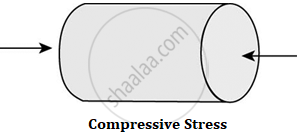2. Tangential or shearing stress:

If deforming force acts tangentially to the surface of a body, it produces a change in the shape of the body. The tangential force applied per unit area is called tangential stress.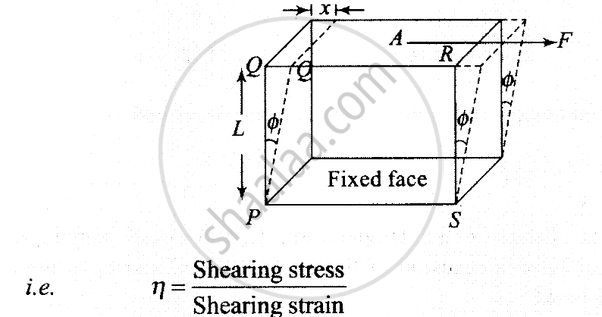3.Normal stress:

If a body is subjected to a uniform force from all sides, then the corresponding stress is called hydrostatic stress.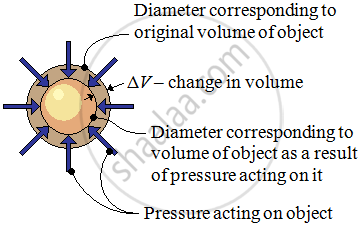Strain:

When a deforming force acts on a body, the body undergoes a change in its shape and size. The fractional change in configuration is called strain.

Strain = change in dimension / original dimension

It has no unit and it is a dimensionless quantity.

Types of strain:

1. Longitudinal strain:

Change in length to the original length of the body due to the longitudinal stress.

• If we apply longitudinal stress to a body either the body elongates or it compresses this change along the length of the body. This change in length is measured by Longitudinal Strain.

• Longitudinal Strain = (Delta"L")/"L"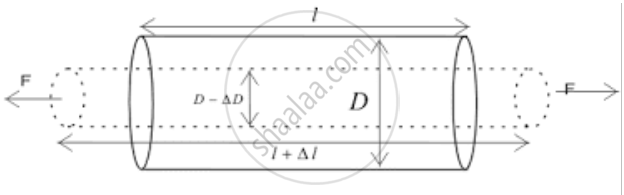• Strain occurs as a result of stress.

2. Volumetric strain:

Volume strain is defined as the ratio of change in volume to the original volume as a result of the hydraulic stress.

• When the stress is applied by a fluid on a body there is a change in the volume of the body without changing the shape of the body.

• Volume strain = "ΔV"/"V"

Therefore, Change in the volume ΔV= V’ – V

• Conclusion: - Deformation is measured using strain.

• Consider a cube whose initial volume is V.

• When the cube is subjected to stress there will be a change in the volume but the shape will not change.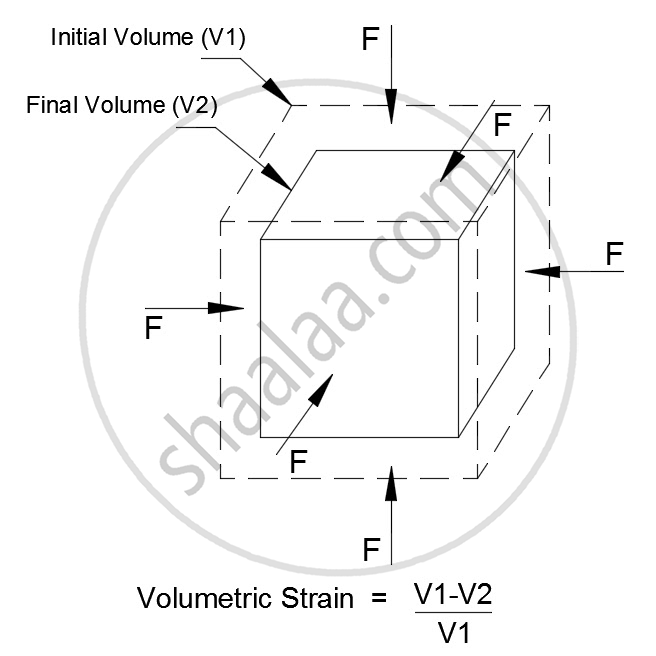• 3. Shearing strain = (Deltax)/"L" = tan theta ... where theta is the angular displacement of the cylinder

Shearing strain is the measure of the relative displacement of the opposite faces of the body as a result of shearing stress.

• If we apply force parallel to the cross-sectional area because of which there was relative displacement between the opposite faces of the body.

• Shearing strain measures to what extent the two opposite faces got displaced relative to each other.

Shearing strain= x/"L"

• In terms of tan θ,

• Shearing strain = tan θ = x/L

• tan θ is equal to θ (as θ is very small)

• Therefore, x/L=theta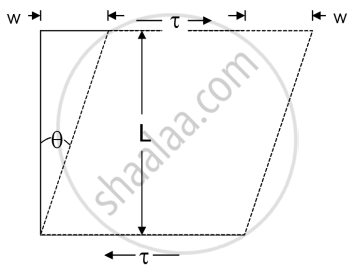If you would like to contribute notes or other learning material, please submit them using the button below.